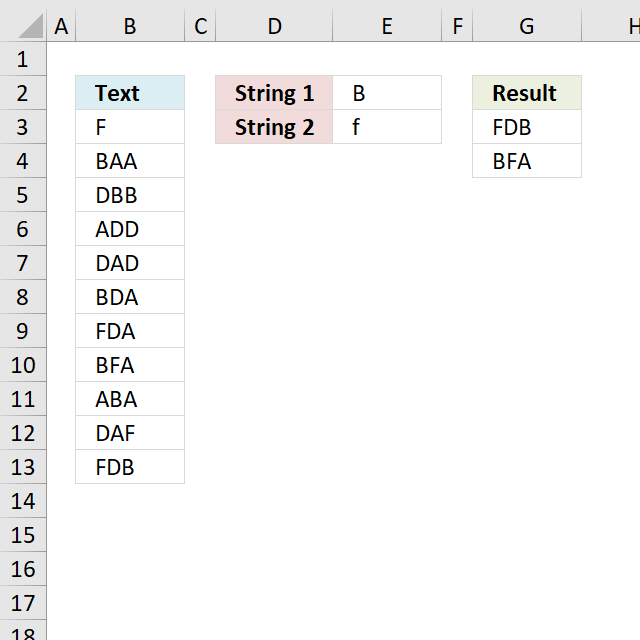Author: Oscar Cronquist Article last updated on November 28, 2018Jerome asks, in this blog post Search for multiple text strings in multiple cells in excel :

If the list of strings in column D was to increase to a large number e.g. 15, how would you tell excel to select the range of strings, so that you don't have to select each string "SEARCH(\$D\$3" in the search parameter, as it seems is the case at the moment?

Array Formula in G3:

=LOOKUP(2, 1/((COUNTIF(\$G\$2:G2, \$B\$3:\$B\$13)=0)*(MMULT(--(ISNUMBER(SEARCH(TRANSPOSE(\$E\$2:\$E\$3), \$B\$3:\$B\$13))), {1; 1})=2)), \$B\$3:\$B\$13)

Change the following in order to add more conditions:

• cell reference \$E\$2:\$E\$3 if you want more conditions
• the number after the second equal sign =2 to as many conditions you have in the formula
• {1; 1} to as many conditions you have. For example, 4 conditions - {1; 1; 1; 1}

#### How to create an array formula

1. Select cell F2
2. Type formula in formula bar3. Press and hold Ctrl + Shift
4. Press Enter
5. Release all keys

### Explaining formula in cell

#### Step 1 - Search for multiple strings

The SEARCH function allows you to find a string in a cell and it's character position. It also allows you to search for multiple strings in multiple cells if you arrange values in a way that works. That is why I use the TRANSPOSE function to transpose the values.

SEARCH(TRANSPOSE(\$E\$2:\$E\$3), \$B\$3:\$B\$13)

becomes

SEARCH(TRANSPOSE({"B";"f"}), \$B\$3:\$B\$13)

becomes

SEARCH({"B","f"}, \$B\$3:\$B\$13)

and returns

{#VALUE!, 1; 1, #VALUE!; 2, #VALUE!; #VALUE!, #VALUE!; #VALUE!, #VALUE!; 1, #VALUE!; #VALUE!, 1; 1, 2; 2, #VALUE!; #VALUE!, 3; 3, 1}

#### Step 2 - Convert values into boolean values

The ISNUMBER function returns TRUE if value is number and FALSE for everything else including errors.

ISNUMBER(SEARCH(TRANSPOSE(\$E\$2:\$E\$3), \$B\$3:\$B\$13))

becomes

ISNUMBER({#VALUE!, 1; 1, #VALUE!; 2, #VALUE!; #VALUE!, #VALUE!; #VALUE!, #VALUE!; 1, #VALUE!; #VALUE!, 1; 1, 2; 2, #VALUE!; #VALUE!, 3; 3, 1})

and returns

{FALSE, TRUE;TRUE, FALSE;TRUE, FALSE;FALSE, FALSE;FALSE, FALSE;TRUE, FALSE;FALSE, TRUE;TRUE, TRUE;TRUE, FALSE;FALSE, TRUE;TRUE, TRUE}

#### Step 3 - Convert boolean values

The MMULT function can't work with boolean values so we need to convert them to their numerical equivalents.

--(ISNUMBER(SEARCH(TRANSPOSE(\$E\$2:\$E\$3), \$B\$3:\$B\$13)))

becomes

--({FALSE, TRUE;TRUE, FALSE;TRUE, FALSE;FALSE, FALSE;FALSE, FALSE;TRUE, FALSE;FALSE, TRUE;TRUE, TRUE;TRUE, FALSE;FALSE, TRUE;TRUE, TRUE})

and returns

{0,1;1,0;1,0;0,0;0,0;1,0;0,1;1,1;1,0;0,1;1,1}.

#### Step 4 - Add numbers in array row-wise

MMULT(--(ISNUMBER(SEARCH(TRANSPOSE(\$E\$2:\$E\$3),\$B\$3:\$B\$13))),{1;1})=2

becomes

MMULT({0,1;1,0;1,0;0,0;0,0;1,0;0,1;1,1;1,0;0,1;1,1}, {1;1})=2

becomes

{1;1;1;0;0;1;1;2;1;1;2}=2

and returns

{FALSE; FALSE; FALSE; FALSE; FALSE; FALSE; FALSE; TRUE; FALSE; FALSE; TRUE}.

#### Step 5 - Prevent duplicates in the list

The next COUNTIF function counts values based on a condition or criteria, the first argument has this cell reference: \$G\$2:G2. It expands as you copy the cell and paste to cells below.

(COUNTIF(\$G\$2:G2, \$B\$3:\$B\$13)=0)

becomes

{0;0;0;0;0;0;0;0;0;0;0}=0

and returns

{TRUE; TRUE; TRUE; TRUE; TRUE; TRUE; TRUE; TRUE; TRUE; TRUE; TRUE}

#### Step 6 - Multiply arrays

We apply AND logic if we multiply the arrays, this means both values must be TRUE in order to return TRUE.

(COUNTIF(\$G\$2:G2, \$B\$3:\$B\$13)=0)* (MMULT(--(ISNUMBER(SEARCH(TRANSPOSE(\$E\$2:\$E\$3), \$B\$3:\$B\$13))), {1; 1})=2)

becomes

{TRUE; TRUE; TRUE; TRUE; TRUE; TRUE; TRUE; TRUE; TRUE; TRUE; TRUE}*{FALSE; FALSE; FALSE; FALSE; FALSE; FALSE; FALSE; TRUE; FALSE; FALSE; TRUE}

and returns

{0;0;0;0;0;0;0;1;0;0;1}

#### Step 6 - Divide 1 with array

The LOOKUP function ignores error values. Divide 1 with zero and we get #DIV/0! error.

1/((COUNTIF(\$G\$2:G2, \$B\$3:\$B\$13)=0)* (MMULT(--(ISNUMBER(SEARCH(TRANSPOSE(\$E\$2:\$E\$3), \$B\$3:\$B\$13))), {1; 1})=2))

becomes

1/{0;0;0;0;0;0;0;1;0;0;1}

and returns

{#DIV/0!; #DIV/0!; #DIV/0!; #DIV/0!; #DIV/0!; #DIV/0!; #DIV/0!; 1; #DIV/0!; #DIV/0!; 1}.

#### Step 7 - Return values

LOOKUP(2, 1/((COUNTIF(\$G\$2:G2, \$B\$3:\$B\$13)=0)*(MMULT(--(ISNUMBER(SEARCH(TRANSPOSE(\$E\$2:\$E\$3), \$B\$3:\$B\$13))), {1; 1})=2)), \$B\$3:\$B\$13)

becomes

LOOKUP(2, {#DIV/0!; #DIV/0!; #DIV/0!; #DIV/0!; #DIV/0!; #DIV/0!; #DIV/0!; 1; #DIV/0!; #DIV/0!; 1}, \$B\$3:\$B\$13)

becomes

LOOKUP(2, {#DIV/0!; #DIV/0!; #DIV/0!; #DIV/0!; #DIV/0!; #DIV/0!; #DIV/0!; 1; #DIV/0!; #DIV/0!; 1}, {"F"; "BAA"; "DBB"; "ADD"; "DAD"; "BDA"; "FDA"; "BFA"; "ABA"; "DAF"; "FDB"})

and returns "FDB" in cell G3.